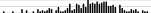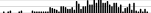Many applications can use the automatically created `data.table` object `.SD` which stands for the data table for the current group being processed. If `.SDcols` were not specified, all variables would be attempted to be analyzed. Specify a vector of variable names as `.SDcols` to restrict the analysis. If there were no `by` variable(s), `.SD` stands for the whole data table.

``````require(Hmisc)
require(data.table)
getHdata(stressEcho)
d <- stressEcho
setDT(d)
# Compute the number of distinct values for all variables
nd <- function(x) length(unique(x))
d[, sapply(.SD, nd)]``````
``````      bhr    basebp    basedp      pkhr       sbp        dp      dose     maxhr
69        94       441       105       142       508         7       103
pctMphr       mbp   dpmaxdo   dobdose       age    gender    baseEF     dobEF
78       132       484         8        62         2        54        60
chestpain   restwma     posSE     newMI   newPTCA   newCABG     death    hxofHT
2         2         2         2         2         2         2         2
hxofDM   hxofCig    hxofMI  hxofPTCA  hxofCABG any.event       ecg
2         3         2         2         2         2         3 ``````
``````# Same but only for variables whose names contain hx and either D or M
d[, sapply(.SD, nd), .SDcols=patterns('hx', 'D|M')]``````
``````hxofDM hxofMI
2      2 ``````
``````# Compute means on all numeric variables
mn <- function(x) mean(x, na.rm=TRUE)
d[, lapply(.SD, mn), .SDcols=is.numeric]``````
``````        bhr   basebp   basedp     pkhr      sbp       dp     dose    maxhr
1: 75.29032 135.3244 10181.31 120.5502 146.9158 17633.84 33.75448 119.3692
pctMphr mbp  dpmaxdo  dobdose      age   baseEF    dobEF chestpain
1: 78.56989 156 18549.88 30.24194 67.34409 55.60394 65.24194 0.3082437
restwma     posSE      newMI   newPTCA    newCABG      death    hxofHT
1: 0.4605735 0.2437276 0.05017921 0.0483871 0.05913978 0.04301075 0.7043011
hxofDM    hxofMI  hxofPTCA  hxofCABG any.event
1: 0.3691756 0.2759857 0.0734767 0.1577061 0.1594982``````
``````# Compute means on all numeric non-binary variables
nnb <- function(x) is.numeric(x) && length(unique(x)) > 2
d[, lapply(.SD, mn), .SDcols=nnb]``````
``````        bhr   basebp   basedp     pkhr      sbp       dp     dose    maxhr
1: 75.29032 135.3244 10181.31 120.5502 146.9158 17633.84 33.75448 119.3692
pctMphr mbp  dpmaxdo  dobdose      age   baseEF    dobEF
1: 78.56989 156 18549.88 30.24194 67.34409 55.60394 65.24194``````
``````# Print frequency tables of all categorical variables with > 2 levels
cmult <- function(x) ! is.numeric(x) && length(unique(x)) > 2
tab <- function(x) {
z <- table(x, useNA='ifany')
paste(paste0(names(z), ': ', z), collapse=', ')
}
d[, lapply(.SD, tab), .SDcols=cmult]``````
``````                                      hxofCig
1: heavy: 122, moderate: 138, non-smoker: 298
ecg
1: normal: 311, equivocal: 176, MI: 71``````

Tabulate all variables having between 3 and 10 distinct values and create a side effect when `data.table` is running that makes the summarization function `tab` store all values and frequencies in a growing list `Z` so that `kable` can render a `markdown` table after we pad columns to the maximum length of all columns (maximum number of distinct values).

``````# Using <<- makes data.table have a side effect of augmenting Z and
# Align in the global environment
tab <- function(x) {
z <- table(x, useNA='ifany')
i <- length(Z)
Z[[i+1]] <<- names(z)
Z[[i+2]] <<- as.vector(z)
Align <<- c(Align, if(is.numeric(x)) 'r' else 'l', 'r')
length(z)
}
discr <- function(x) { i <- length(unique(x)); i > 2 & i < 11 }
#                                       or i %between% c(2,11)
Z    <- list(); Align <- character(0)
w    <- d[, lapply(.SD, tab), .SDcols=discr]
maxl <- max(w)
# Pad shorter vectors with blanks
Z <- lapply(Z, function(x) c(x, rep('', maxl - length(x))))
Z <- do.call('cbind', Z)  # combine all into columns of a matrix
colnames(Z) <- rep(names(w), each=2)
colnames(Z)[seq(2, ncol(Z), by=2)] <- 'Freq'
knitr::kable(Z, align=Align)``````
dose Freq dobdose Freq hxofCig Freq ecg Freq
10 2 5 7 heavy 122 normal 311
15 28 10 7 moderate 138 equivocal 176
20 47 15 55 non-smoker 298 MI 71
25 56 20 73
30 64 25 71
35 61 30 78
40 300 35 62
40 205

A better approach is to let the `kables` function put together a series of separate `markdown` tables of different sizes. By using the “updating `Z` in the global environment” side effect we are able to let `data.table` output any type of objects of non-conformable dimensions over variables (such as frequency tabulations).

``````tab <- function(x) {
z <- table(x, useNA='ifany')
i <- length(Z)
w <- matrix(cbind(names(z), z), ncol=2,
dimnames=list(NULL, c(vnames[i+1], 'Freq')))
Z[[i+1]] <<- knitr::kable(w, align=c(if(is.numeric(x)) 'r' else 'l', 'r'))
length(z)
}
discr <- function(x) { i <- length(unique(x)); i > 2 & i < 11 }
Z      <- list()
vnames <- names(d[, .SD, .SDcols=discr])
w      <- d[, lapply(.SD, tab), .SDcols=discr]
knitr::kables(Z)``````
dose Freq
10 2
15 28
20 47
25 56
30 64
35 61
40 300
dobdose Freq
5 7
10 7
15 55
20 73
25 71
30 78
35 62
40 205
hxofCig Freq
heavy 122
moderate 138
non-smoker 298
ecg Freq
normal 311
equivocal 176
MI 71

Use a similar side-effect approach to get separate `html` `describe` output by `gender`.

``````g <- function(x, by) {
Z[[length(Z) + 1]] <<- describe(x, descript=paste('age for', by))
by
}
Z <- list()
by <- d[, g(age, gender), by=gender]
# Make Z look like describe() output for multiple variables
class(Z) <- 'describe'
attr(Z, 'dimensions') <- c(nrow(d), nrow(by))
attr(Z, 'descript') <- 'Age by Gender'
html(Z)``````
Age by Gender Descriptives
Age by Gender

2 Variables   558 Observations

age for male: Age yearsnmissingdistinctInfoMeanGmd.05.10.25.50.75.90.95
2200510.99967.8612.9145.9551.0062.0069.0075.0081.0086.00
lowest : 30 34 38 40 43 , highest: 88 89 91 92 93
age for female: Age yearsnmissingdistinctInfoMeanGmd.05.10.25.50.75.90.95
3380580.99967.0113.7447.0050.7059.2568.0076.0083.0085.00
lowest : 26 28 29 33 34 , highest: 87 88 89 90 91
``````# Compute a 1-valued statistic on multiple variables, by cross-classification
# of two variables.  Do this on a subset.  .SDcols=a:b uses variables in order
# Use keyby instead of by to order output the usual way
d[age < 70, lapply(.SD, mean), keyby=.(gender, newMI), .SDcols=pkhr:dp]``````
``````   gender newMI     pkhr      sbp       dp
1:   male     0 122.0561 147.7664 17836.24
2:   male     1 115.6667 140.6667 16437.67
3: female     0 122.8492 150.5084 18509.03
4: female     1 123.5714 171.5714 21506.71``````
``````# Compute multiple statistics on one variable
# Note: .N is a special variable: count of obs for current group
d[, .(Max=max(bhr), Min=min(bhr), Mean=mean(bhr), N=.N), by=.(gender, newMI)]``````
``````   gender newMI Max Min     Mean   N
1:   male     0 127  42 74.15385 208
2:   male     1  89  59 71.25000  12
3: female     0 210  45 75.96894 322
4: female     1 108  65 79.43750  16``````
``````# Same result another way
g <- function(x) list(Max=max(x), Min=min(x), Mean=mean(x), N=length(x))
d[, g(bhr), by=.(gender, newMI)]  # if g returned a vector instead, use as.list(g(bhr))``````
``````   gender newMI Max Min     Mean   N
1:   male     0 127  42 74.15385 208
2:   male     1  89  59 71.25000  12
3: female     0 210  45 75.96894 322
4: female     1 108  65 79.43750  16``````
``d[, as.list(quantile(bhr)), by=gender]``
``````   gender 0% 25% 50% 75% 100%
1:   male 42  63  72  83  127
2: female 45  65  75  85  210``````
``````# Compute mean bhr by quintiles of age using Hmisc::cut2
d[, .(Mean=mean(bhr)), keyby=.(fifth=cut2(age, g=5))]``````
``````     fifth     Mean
1: [26,60) 78.54545
2: [60,67) 76.96907
3: [67,72) 74.26316
4: [72,78) 75.92793
5: [78,93] 70.03846``````
``````# Compute multiple statistics on multiple variables
d[, lapply(.SD, quantile), by=gender, .SDcols=.q(bhr, pkhr, sbp)]``````
``````    gender bhr   pkhr    sbp
1:   male  42  52.00  80.00
2:   male  63 106.75 120.00
3:   male  72 123.00 140.00
4:   male  83 136.00 166.00
5:   male 127 176.00 250.00
6: female  45  61.00  40.00
7: female  65 106.25 122.25
8: female  75 122.00 141.50
9: female  85 134.00 170.00
10: female 210 210.00 309.00``````
``````# Similar but put percentile number in front of statistic value
# Do only quartiles
g <- function(x) {
z <- quantile(x, (1:3)/4, na.rm=TRUE)
paste(format(names(z)), format(round(z)))
}
d[, lapply(.SD, g), by=gender, .SDcols=.q(bhr, pkhr, sbp)]``````
``````   gender    bhr    pkhr     sbp
1:   male 25% 63 25% 107 25% 120
2:   male 50% 72 50% 123 50% 140
3:   male 75% 83 75% 136 75% 166
4: female 25% 65 25% 106 25% 122
5: female 50% 75 50% 122 50% 142
6: female 75% 85 75% 134 75% 170``````
``````# To have more control over labeling and to have one row per sex:
g <- function(x) {
s <- sapply(x, quantile, na.rm=TRUE)  # compute quantiles for all variables -> matrix
h <- as.list(s)  # vectorizes first
# Cross row names (percentile names) with column (variable) names
# paste(b, a) puts variable name in front of percentile
names(h) <- outer(rownames(s), colnames(s), function(a, b) paste(b, a))
h
}
d[, g(.SD), by=gender, .SDcols=.q(bhr, pkhr, sbp)]``````
``````   gender bhr 0% bhr 25% bhr 50% bhr 75% bhr 100% pkhr 0% pkhr 25% pkhr 50%
1:   male     42      63      72      83      127      52   106.75      123
2: female     45      65      75      85      210      61   106.25      122
pkhr 75% pkhr 100% sbp 0% sbp 25% sbp 50% sbp 75% sbp 100%
1:      136       176     80  120.00   140.0     166      250
2:      134       210     40  122.25   141.5     170      309``````
``````# Restrict to variables bhr - basedp in order columns created in data table
d[, g(.SD), by=gender, .SDcols=bhr : basedp]``````
``````   gender bhr 0% bhr 25% bhr 50% bhr 75% bhr 100% basebp 0% basebp 25%
1:   male     42      63      72      83      127        85     120.00
2: female     45      65      75      85      210        90     122.25
basebp 50% basebp 75% basebp 100% basedp 0% basedp 25% basedp 50% basedp 75%
1:        130        145         203      5220    7976.25       9483   11183.50
2:        136        150         201      5000    8562.00      10063   11891.25
basedp 100%
1:       16704
2:       27300``````
``````# Can put ! in front of a sequence of variables to do the opposite

# To add duplicated means to raw data use e.g.
# d[, Mean := mean(x), by=sex]``````

## 10.1 Summary Statistics With Marginal Summaries

The `cube` function in the `data.table` package will compute all possible marginal statistics. When there is only one `by` variable, the overall statistic is computed in addition to compute stratified values. When a dimension is being marginalized over, the value of the `by` variable for that dimension will be `NA`.

``````mn  <- function(x) as.double(mean(x, na.rm=TRUE))
# as.double ensures consistency of numeric type across groups
Nna <- function(x) sum(! is.na(x))
cube(d, .(Meanbhr = mn(bhr), N = Nna(bhr)), by='gender', id=TRUE)``````
``````   grouping gender  Meanbhr   N
1:        0   male 73.99545 220
2:        0 female 76.13314 338
3:        1   <NA> 75.29032 558``````
``cube(d, .(Meanbhr = mn(bhr), N = Nna(bhr)), by=.q(gender, hxofMI), id=TRUE)``
``````   grouping gender hxofMI  Meanbhr   N
1:        0   male      1 73.22472  89
2:        0 female      0 76.30403 273
3:        0   male      0 74.51908 131
4:        0 female      1 75.41538  65
5:        1   male     NA 73.99545 220
6:        1 female     NA 76.13314 338
7:        2   <NA>      1 74.14935 154
8:        2   <NA>      0 75.72525 404
9:        3   <NA>     NA 75.29032 558``````
``````# id=TRUE creates output variable grouping to detail level of marginalization
# It is a binary representation, e.g. if by has 3 variables and a row
# is marginalizing over the first and third variables,
# grouping=binary 101 = 5
# Use groupingsets() to control the marginalizations
# Example: marginalize only one variable at a time
groupingsets(d, .(Meanbhr = mn(bhr), N=Nna(bhr)), by=.q(gender, hxofMI),
sets=list('gender', 'hxofMI'), id=TRUE)``````
``````   grouping gender hxofMI  Meanbhr   N
1:        1   male     NA 73.99545 220
2:        1 female     NA 76.13314 338
3:        2   <NA>      1 74.14935 154
4:        2   <NA>      0 75.72525 404``````